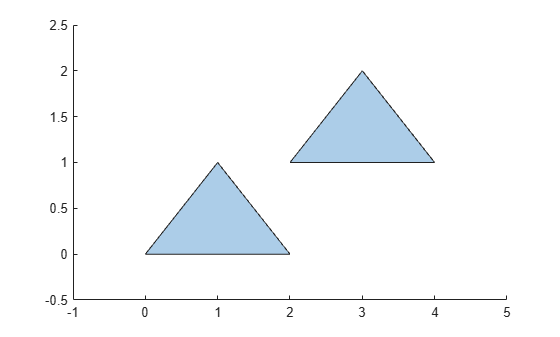# boundary

Vertex coordinates of `polyshape` boundary

## Syntax

``[x,y] = boundary(polyin)``
``[x,y] = boundary(polyin,I)``

## Description

example

````[x,y] = boundary(polyin)` returns the x-coordinates and the y-coordinates of each boundary of a `polyshape`. The vectors `x` and `y` contain the list of coordinates for each boundary, delimited by `NaN`. The `boundary` function automatically appends the first vertex coordinates of `polyin` to the end of `x` and `y` to close the polygon.```
````[x,y] = boundary(polyin,I)` returns the coordinates of the `I`th boundary of a `polyshape`.```

## Examples

collapse all

Create a polygon containing two boundaries, and display the vertex coordinates of both boundaries. The list of vertices for the boundaries are delimited by `NaN`.

```x1 = [0 1 2]; y1 = [0 1 0]; x2 = [2 3 4]; y2 = [1 2 1]; polyin = polyshape({x1,x2},{y1,y2}); plot(polyin)````[x,y] = boundary(polyin)`
```x = 9×1 0 1 2 0 NaN 2 3 4 2 ```
```y = 9×1 0 1 0 0 NaN 1 2 1 1 ```

## Input Arguments

collapse all

Input `polyshape`, specified as a scalar.

Data Types: `polyshape`

Boundary index, specified as a scalar integer or vector of integers. Each element of `I` corresponds to a single boundary of the input `polyshape`.

Data Types: `double` | `single` | `int8` | `int16` | `int32` | `int64` | `uint8` | `uint16` | `uint32` | `uint64`

## Output Arguments

collapse all

x-coordinates of `polyshape` boundaries, returned as a column vector. When returning multiple boundaries, `boundary` places `NaN` values between each boundary's set of coordinates.

Data Types: `double`

y-coordinates of `polyshape` boundary, returned as a column vector. When returning multiple boundaries, `boundary` places `NaN` values between each boundary's set of coordinates.

Data Types: `double`

## Version History

Introduced in R2017b